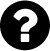## Michael Grünstäudl (Gruenstaeudl), PhD

### Postdoctoral Researcher at the Freie Universität Berlin## State-matrix to presence-absence-matrix

A quick R example

Today, I needed to convert a series of state matrices into presence-absence matrices. In order to automate this conversion, I wrote the following R code. (The initiated will recognize the output as a species-range matrix.)

1.a. Generate example input

```m = matrix(data=c("A","B","C"), nrow=3, ncol=1) rownames(m) = c("t1", "t2", "t3") m```
[,1]
t1 “A”
t2 “B”
t3 “C”

1.b. Alternatively, one could load a table from file

```t = as.matrix(read.csv("~/test.csv", header=FALSE)) m = as.matrix(t[,2]) rownames(m) = t[,1] ```

2. Generate the presence-absence matrix

```l = length(unique(m[,1])) m = cbind(m, matrix(rep(0,l), nrow=nrow(m), ncol=l)) colnames(m)=c(NA,c(unique(m[,1]))) for (i in c(unique(m[,1]))) {m[which(m[,1] %in% i), i]=1} m = m[,-1] m```
A B C
t1 “1” “0” “0”
t2 “0” “1” “0”
t3 “0” “0” “1”

EDIT: If any of the entries in the state matrix were to contain a combination of states (e.g., “A and B”), the following code should be used at step #2:

```tmp = unique(m[,1]) new = tmp[-which(tmp==tmp[grepl(" and ", tmp)])] addon = unlist(strsplit(tmp[grepl(" and ", tmp)], ' and ')) ul = unique(c(new,addon)) l = length(ul) m = cbind(m, matrix(rep(0,l), nrow=nrow(m), ncol=l)) colnames(m)=c(NA,ul) for (i in ul) {m[which(grepl(i, m[,1])), i]=1} m = m[,-1] ```

##### Der Beitrag wurde am Tuesday, den 25. August 2015 um 17:20 Uhr von Michael Grünstäudl veröffentlicht und wurde unter bioinformatics abgelegt. Sie können die Kommentare zu diesem Eintrag durch den RSS 2.0 Feed verfolgen. Sie können einen Kommentar schreiben, oder einen Trackback auf Ihrer Seite einrichten.Hinweis / Hint
Das Captcha kann Kleinbuchstaben, Ziffern und die Sonderzeichzeichen »?!#%&« enthalten.
The captcha could contain lower case, numeric characters and special characters as »!#%&«.